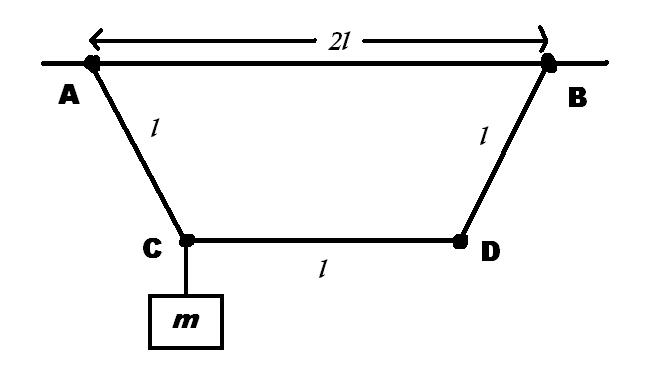# Minimum Force

Three weightless rods of length $l$ each are hinged at point $A$ and $B$ lying on the same horizontal and joint through hinges at points $C$ and $D$ (shown below).The length $A$$B$ $=$ $2$$l$. A load of mass $m$ is suspended at hinge $C$.

Determine the minimum force $F$ applied to the hinge $D$ for which the middle rod remains horizontal.

If $F$ can be described as $\dfrac{mg}{k}$, determine $k$

×